# On r-dynamic coloring of comb graphs

K. Kalaiselvi, N. Mohanapriya and J. Vernold Vivin
Notes on Number Theory and Discrete Mathematics
Print ISSN 1310–5132, Online ISSN 2367–8275
Volume 27, 2021, Number 2, Pages 191—200
DOI: 10.7546/nntdm.2021.27.2.191-200

## Details

### Authors and affiliations

K. KalaiselviDepartment of Mathematics, Dr. Mahalingam College of Engineering and Technology

N. MohanapriyaPG and Department of Mathematics, Kongunadu Arts and Science College

J. Vernold VivinDepartment of Mathematics, University College of Engineering Nagercoil
(A Constituent College of Anna University, Chennai)
Konam, Nagercoil-629 004, Tamil Nadu, India

### Abstract

An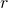-dynamic coloring of a graph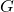is a proper coloring ofsuch that every vertex in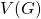has neighbors in at least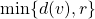different color classes. The-dynamic chromatic number of graphdenoted as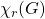, is the least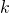such thathas a coloring. In this paper we obtain the-dynamic chromatic number of the central graph, middle graph, total graph, line graph, para-line graph and sub-division graph of the comb graphdenoted by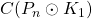,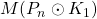,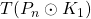,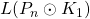,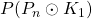and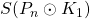respectively by finding the upper bound and lower bound for the-dynamic chromatic number of the Comb graph.

### Keywords

• r-dynamic coloring
• Comb graph
• Central graph
• Middle graph
• Total graph
• Line graph
• Sub-division graph
• Para-line graph

• 05C15
• 05C75

### References

1. Adegoke, K. (2018). Partial sums and generating functions for power of second order sequences with indices in arithmetic progression. Preprint. Available online at: https://arxiv.org/abs/1904.09916.
2. Belbachir, H., & Bencherif, F. (2013). Sums of products of generalized Fibonacci and Lucas numbers. Ars Combinatoria, 110, 33–43.
3. Čerin, Z. (2015). Formulas for quadratic sums that involve generalized Fibonacci and Lucas numbers. Rad Hrvatske Akademije Znanosti i Umjetnosti, Matematicke Znanosti, 19(523), 1–12.
4. Čerin, Z. (2009). On sum of products of Horadam numbers. Kyungpook Mathematical Journal, 49, 483–492.
5. Čerin, Z. (2009) Sum of products of generalized Fibonacci and Lucas numbers.
Demonstratio Mathematica, 42, 247–258.
6. Horadam, A. F. (1965). Basic properties of a certain generalized sequence of numbers. The Fibonacci Quarterly, 3(3), 161–176.
7. Khan, M. A., & Kwong, H. (2014). On sums of products of Fibonacci-type recurrences. The Fibonacci Quarterly, 52(2), 20–26.
8. Kiliç, E., Ömür, N., & Ulutas, Y. T. (2011). Some finite sums involving generalized Fibonacci and Lucas numbers. Discrete Dynamics in Nature and Society, 2011(1), Article ID 284261.
9. Kiliç, E., & Prodinger, H. (2015). Sums of products of generalized Fibonacci and Lucas numbers. Acta Mathematica Hungarica, 145(1), 17–25.
10. Kiliç, E., & Stănică, P. (2013). General approach in computing sums of products of binary sequences. Hacettepe Journal of Mathematics and Statistics, 42(1), 1–7.
11. Koshy, T. (2017). Fibonacci and Lucas Numbers with Applications, Wiley, New York.
12. Koshy, T. (2014). Pell and Pell–Lucas Numbers with Applications, Springer, New York.
13. Larcombe, P. J. (2017). Horadam sequences: A survey update and extension. Bulletin of the Institute of Combinatorics and its Applications, 80, 99–118.
14. Ribenboim, P. (2000). My Numbers, My Friends, Springer, New York.
15. Sloane, N. J. A. (ed.). The On-Line Encyclopedia of Integer Sequences. Available online at: https://oeis.org.
16. Vajda, S. (2008). Fibonacci & Lucas Numbers, and the Golden Section: Theory and Applications, Dover, New York.

## Cite this paper

Kalaiselvi, K., Mohanapriya, N., & Vernold Vivin, J. (2021). On r-dynamic coloring of comb graphs. Notes on Number Theory and Discrete Mathematics, 27(2), 191-200, doi: 10.7546/nntdm.2021.27.2.191-200.